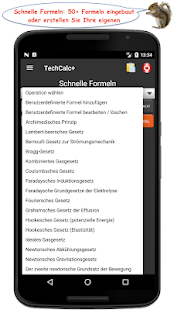TechCalc+ scientific calculator contains 12 calculation modes in one application + a handy reference section that includes an additional 22 calculation modes plus a wealth of useful information.

Perfect for all aspects of science and engineering calculations in school, university and throughout your career. Why not download it now and give it a try?

Modes included are:

★ Basic Mode,
★ Scientific Mode,
★ 64-bit Programmer Mode (Hex, Oct, Bin and Dec),
★ Graphing,
★ Matrices,
★ Complex Numbers (cartesian, polar, using Euler’s identity),
★ Quick Formulas,
★ Quick Converter,
★ Time Calculator,
★ Equation Solver,
★ Calculus (Derivatives, Definite Integrals, Taylor Series, Indefinite Integrals & Limits)
★ Financial

+ the Periodic Table of Elements!

Features include:
★ Powers & Roots
★ Logs and Antilogs
★ Factorial, Modulus & Random Numbers functions
★ HCF, LCM, Prime factorization
★ Pol() & Rec() Functions
★ Permutations (nPr) and Combinations (nCr)
★ Statistics
★ Fractions Mode
★ A wide range of conversion categories and constants
★ 20 Memory Registers in each of the calculation modes
★ Detailed calculation history
★ Extensive Help and Reference
★ Highly customizable via the Settings

The reference section includes:
★ Physical Laws
★ Names in the Metric System
★ Mathematical Tables
★ Elementary & Linear Algebra
★ Trigonometric Identities
★ Differentiation & Integration Rules
★ Statistics Formulas
★ Vector Mathematics
★ ASCII, Fractional Bits, Roman Numeral & Number Base Converters
★ pH, Interpolation, Body Mass Index (BMI), Percentage, Proportion & Molecular Weight Calculators
★ Sigma & Pi Notation
★ Balancing Chemical Equations
★ Statistics (Grouped Data)
★ Numerical Sequences
★ Humidity Calculations
★ Boolean Algebra Calculator
★ Empirical Formula Calculator
★ Characteristics of an RLC Circuit
★ Feet and Inches Calculator
★ Aspect Ratio Calculator
★ Barometric Formula Calculator
★ Linear Regression Analysis (Simple Linear Regression; Multiple Linear Regression)

Whats New:
ver 4.4.8:
★ added ability to do simple calculations on the main screen of Financial mode
★ added an option in the “Calculation Parameters” group of the Settings: Financial – Keep trailing zeros in simple calculations
★ added an option in the “Screen Modes” group of the Settings: Swipe Screen – Arrange Calculator List. Please see the topic “Screen Transitions” in the Help Section for details

Screenshots

•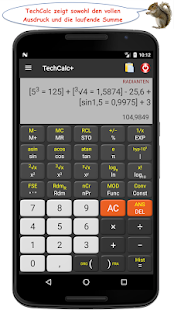•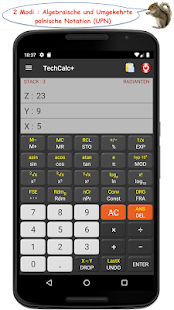•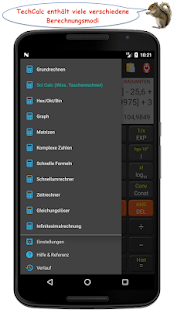•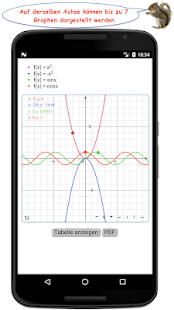•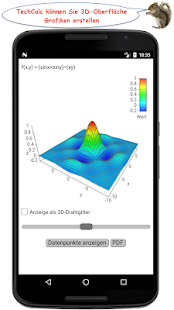••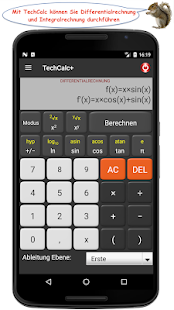•